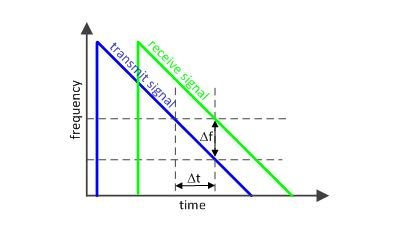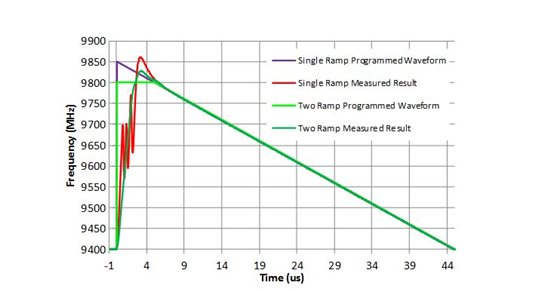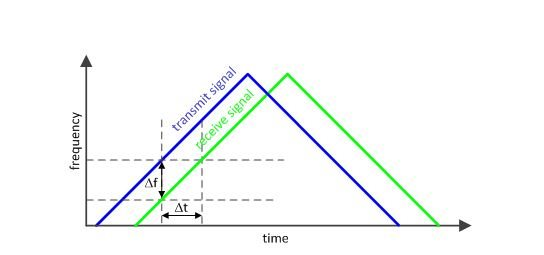# Timing is Everything: How to create modulated waveforms using fractional PLLs

We’ve likely all seen situations where we’ve needed to sweep the frequency over time.  If you are faced with this, consider an application like radar where a transmitted signal is bounced off a target and compared to the received signal, as shown below in Figure 1.  If we look at the difference in frequency (Df), we can determine the time that the signal took to return (Dt). Once this time is known, we can then find the distance to the target.   If the slope of the line is made steeper, then the system is less sensitive to noise, but this comes at the expense of reducing the range.Figure 1: Frequency chirp waveform

For radar applications, it is important that the waveform produced in Figure 1 is very linear and has a constant slope in order to avoid frequency miscalculation.  In applications where more nonlinearity can be tolerated, use a digital to analog converter (DAC) to steer the control voltage of a voltage controlled oscillator (VCO) in order to generate the desired waveform. One challenge with this open loop approach is that the slope of the waveform is impacted by part-to-part variations, temperature, VCO frequency drift and VCO frequency supply pushing.

For applications demanding better linearity, an alternative method is a Phased Locked Loop (PLL), like the LMX2492, to create the waveform by adding fractional modulation in the feedback divider.   Figure 2 below shows an actual measurement that emphasizes one of the challenges for a 45us frequency chirp from 9850 to 9400 MHz.   The abrupt frequency change causes overshoot and cycle slipping, but this is reduced by programming the device to 9800 MHz and staying there for 5us and then continuing the ramp.  By using this two ramp approach, as shown in Figure 2, performance is improved.Figure 2: Measured frequency chirp with the LMX2492

Aside from using additional frequency ramps to improve the linearity of the waveform, they can also be used to create more complicated waveforms.  For instance, a dual ramp approach, as shown below in Figure 3, can be used to account for the Dopper shift for a moving target.Figure 3: Dual ramp approach

The examples I’ve shown are fairly basic, but you can create much more complicated waveforms using more linear segments, or to introduce more ramps.